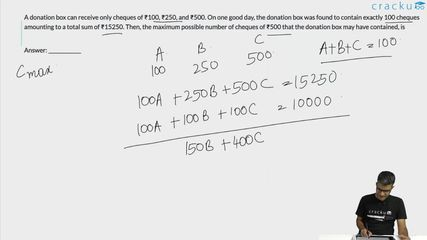Question 63

# A donation box can receive only cheques of ₹100, ₹250, and ₹500. On one good day, the donation box was found to contain exactly 100 cheques amounting to a total sum of ₹15250. Then, the maximum possible number of cheques of ₹500 that the donation box may have contained, is

Solution

Let the number of 100 cheques, 250 cheques and 500 cheques be x, y and z respectively.

We need to find the maximum value of z.

x + y + z = 100 ...... (1)

100x + 250y + 500z = 15250

2x + 5y + 10z = 305 ...... (2)

2x + 2y + 2z = 200 ....... (1)

(2) - (1), we get

3y + 8z = 105

At z = 12, x = 3

Therefore, maximum value z can take is 12.

### View Video Solution• All Quant CAT Formulas and shortcuts PDF
• 30+ CAT previous papers with solutions PDF

##### aayush goel

2 months, 4 weeks ago

Why cant we take the 250 out of 15250 and get 30 500rs cheque as it is no where mentioned that you need to have a cheque of 100rs clearly mentioned that the mail box can recieve

##### Pandyan Quest

2 weeks, 2 days ago

number of cheques is 100. in this case it is 31 so it doesnt match the condition

##### Divyansh

1 month ago

I thought the same

##### Pikachu Gamer

2 months, 1 week ago

Sir it said maximum possible value right so 500 notes can be 29 and 5 100 notes and 1 250 note this case is also possible then 29 must be the answer righttt???